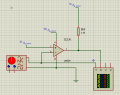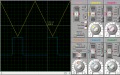# LM339 as PWM issue

#### Creation Opj

Joined Jul 3, 2019
8
Hi. I wonder if anyone can help me to understand this issue:

So I was testing the Non Inverting PWM and the Inverting PWM for this op amp. I'm generating a triangle wave with 10Vpp and F=100hz

CIRCUIT:For the Inverting: I calculated and manually plotted the graph of Vo VS t, and then I simulated with Proteus to check if I was correct, and indeed I was.
For the Non inverting: I also plotted this graph, and the results I had where theses ones:

When the AC signal reaches 3v and 5v, then Vo=5v, which means TH= 3ms.
But when I simulated for the Non Inverting input, I've got a different graph:As you can see, it happens that the values for Vo are inverted, and Vo is HIGH when the triangle wave reaches negative values of -2 and -5, the signal stays in HIGH 3ms, but the values for Vo are not the same and according to this statement where:

Ed= V+ (-) V- If Ed's positive then Vo=HIGH, if Ed's negative then Vo=LOW

Then according to that:
(1) Ed = -5-3 = -8 LOW
(2) Ed = -4-3= -7 LOW
(3) Ed = -3-3= -6 LOW
(4) Ed = -2-3= -5 LOW
(5) Ed = -1-3= -4 LOW
(6) Ed = 0-3= -3 LOW
(7) Ed = 1-3= -2 LOW
(8) Ed = 2-3 = -1 LOW
(9) Ed = 3-3 = 0 LOW
(10) Ed = 4-3 = 1 HIGH
(11) Ed = 5-3 = 1 HIGH

Vo should be LOW for all those values except for (10) and (11), right?
If I'm wrong, then what could be I missing? I've been reading about this but can't find a clear answer.

If somebody can help me, I'll be extremely grateful!

#### ericgibbs

Joined Jan 29, 2010
16,031
hi CO,
Welcome to AAC.
Are you saying you expect the comparator switching level/point to be the same for the INV and NI inputs.?

Is the triangular waveform input +10Vpp about 0V or above 0V. ?

E

#### Creation Opj

Joined Jul 3, 2019
8
Hi, thanks!

No, I don't expect them to be the same. I know the inverting has a different duty cycle than the non inverting.

The 10vpp is about 0v which means I have 5v & -5v amplitude
T= 10ms
F= 100

#### Jony130

Joined Feb 17, 2009
5,405
For the circuit shown on the diagram for any Vin voltage lower than 3V, you should get a HIGH at the output.
Also, notice that the voltage at the input should never go below GND.

•Creation Opj

#### crutschow

Joined Mar 14, 2008
30,458
When operating the LM339 from a single supply, the input signal should never go negative.

•Creation Opj

#### ericgibbs

Joined Jan 29, 2010
16,031
hi CO,
This plot is for dual supplies to the LM339 of +/12v
E

#### Attachments

•Creation Opj

#### Creation Opj

Joined Jul 3, 2019
8
For the circuit shown on the diagram for any Vin voltage lower than 3V, you should get a HIGH at the output.
Also, notice that the voltage at the input should never go below GND.
Oops, that was the diagram for the Inverting circuit, the graph shown on my post was for the non inverting circuit which basically is the same but with inverted inputs.

When operating the LM339 from a single supply, the input signal should never go negative.
I completely forgot about this! So that means the whole circuit is wrong, I cant design this circuit if I am only using a single supply.

hi CO,
This plot is for dual supplies to the LM339 of +/12v
E
This makes more sense, and this is exactly what I got when I calculated an plotted manually. I was using wrong values when simulating.

Thank you all for your time! You helped me a lot!

#### ericgibbs

Joined Jan 29, 2010
16,031
ericgibbs said:
For the circuit shown on the diagram for any Vin voltage lower than 3V, you should get a HIGH at the output.
Also, notice that the voltage at the input should never go below GND.
hi,
I cannot take the Credit for that, it was @Jony130 ...E